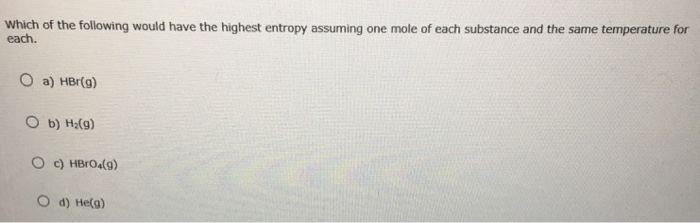Chemistry Practice Problems Entropy Practice Problems Solution: Which of the following would have the highest entr...

# Solution: Which of the following would have the highest entropy assuming one mole of each substance and the same temperature for each. a) HBr(g) b) H2(g) c) HBrO4 d) He(g)

###### Problem

Which of the following would have the highest entropy assuming one mole of each substance and the same temperature for each.

a) HBr(g)

b) H2(g)

c) HBrO

d) He(g)###### Solution

Entropy can be related to # atoms present in the moleculeView Complete Written Solution

Entropy

Entropy

#### Q. Consider the following reaction at 298 ºK: C(graphite) + 2Cl2(g)→CCl4(l) Δ H = -139 kJ Calculate the following quantities. Find standard entropy value...

Solved • Sun Apr 29 2018 13:36:49 GMT-0400 (EDT)

Entropy

#### Q. Given the values of ΔHºrxn, ΔSºrxn, and T below determine ΔSuniv.ΔHºrxn = -81 kJ,ΔSrxn = -149 J/K,T = 301 ºKExpress your answer using two significant ...

Solved • Wed Apr 18 2018 15:03:41 GMT-0400 (EDT)

Entropy

#### Q. For one mole of substance at a given temperature, select the member in each pair with the higher entropy.a. P2(g), P4(g)b. CaF2(s), BaCl2(s)c. CuSO4(s...

Solved • Fri Mar 30 2018 14:54:02 GMT-0400 (EDT)

Entropy

#### Q. Consider the reaction:3 Fe2O3 (s) + H2 (g) → 2Fe3O4 (s) + H2O(g)Using standard absolute entropies at 298K, calculate the entropy change for the system...

Solved • Fri Mar 30 2018 14:27:48 GMT-0400 (EDT)